# Examples On Conditions On Roots Of Quadratics

Example - 21

Let $$\alpha ,\beta$$  be the roots of $${x^2} - \left( {m - 3} \right)x + m = 0.$$ Find the values of m for which:

 (a) the roots are real (b)  $$\alpha ,\beta$$  are of opposite sign (c)  $$\alpha ,\beta$$  are both positive (d)  at least one of the roots is positive (e) $$\alpha > 2,\,\beta < 2$$ (f)  $$\alpha ,\beta$$  both greater than 2 (g) $$\alpha ,\beta$$ lie in (1, 2) (h) exactly one of the roots lies in (1, 2)

Solution: We will be directly using the results derived in this section. Readers are advised to refer to these results as we solve the questions above:

(a) For real roots,

\begin{align}&D \ge 0\\\ \Rightarrow\quad & {\left( {m - 3} \right)^2} - 4m \ge 0\\\Rightarrow\quad & {m^2} - 10m + 9 \ge 0\\\Rightarrow\quad & \left( {m - 1} \right)\left( {m - 9} \right) \ge 0\\ \Rightarrow\quad & m \le 1\,\,{\rm{or}}\,\,m \ge 9\end{align}

(b) Since  $$\alpha ,\beta$$ are of opposite sign,

\begin{align}\frac{c}{a} < 0\\ \Rightarrow \quad m < 0\end{align}

(c)   We have three constraints for this case

\begin{align}&{b^2} - 4ac \ge 0 \;\;\;\; \Rightarrow \;\;\;\; m \le 1\,{\rm{or}}\,m \ge 9 \;\;\;\; \left( {{\rm{Part}}\left( {\rm{a}} \right){\rm{above}}} \right)\\&\;\;\;\;\;\;\;\frac{{ - b}}{a} > 0 \;\;\;\; \Rightarrow \;\;\;\; m - 3 > 0 \;\;\;\; \Rightarrow \;\;\;\; m > 3\\&\;\;\;\;\;\;\;\;\;\frac{c}{a} > 0 \;\;\;\; \Rightarrow \;\;\;\; m > 0\end{align}

The required values for m will be those that satisfy all the three constraints above. We can find those values by drawing the solution sets corresponding to the three inequalities on a real line and then determining their intersections: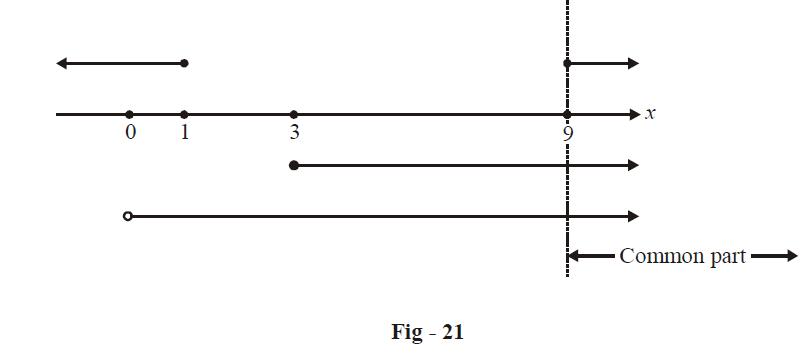We see that the intersection (common part) for the three sets is

$$m \ge 9$$

or $$m \in [9,\infty )$$

(d) We require at least one of the roots to be positive. This means that both the roots cannot be negative.

Hence, what we can do is first evaluate the values of m for which both roots are negative (or one  root is zero and the other is negative), and then exclude these values from the set $$\left( { - \infty ,1]\, \cup \,[9,\infty } \right)$$  {the set for which the roots are real}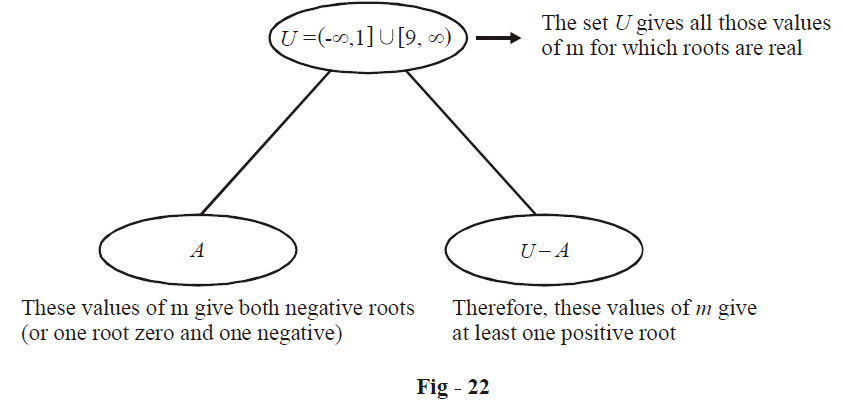To evaluate the set A (the values of m which both the roots are negative),

we require (in addition to $$D \ge 0$$ ):

\begin{align}&\frac{{ - b}}{a} < 0 \quad\Rightarrow \quad m - 3 < 0 \quad \Rightarrow \quad m < 3\\ &\frac{c}{a} \geqslant 0 \quad\;\;\Rightarrow\quad m \geqslant 0 \\ \end{align}

Therefore, the set A is [0, 1] (verify this by finding the intersection $$\left( {m < 3} \right) \cap \left( {m \ge 0} \right) \cap \left( {{\rm{the}}\,{\rm{set}}\,\,U} \right)$$

Finally, the required values of m are UA

or  $$m \in \left( { - \infty ,0)\,\, \cup \,\,[9,\infty } \right)$$

Alternatively, we could also have solved this part by using the solutions to (part-b) and  (part-c)

At least one positive root implies either both roots positive $$\left( {m \ge 9} \right)$$ or one root positive and one negative (roots of opposite sign; m < 0).

Therefore, either $$m \ge 9\,\,{\rm{or}}\,\,m < 0$$

$m \in \left( { - \infty ,0)\,\, \cup \,\,[9,\infty } \right)$

(e) This requirement corresponds to the case “roots lie on either side of k”.

We require

\begin{align}&af\left( k \right) < 0\\&1 \cdot \left\{ {{{\left( 2 \right)}^2} - \left( {m - 3} \right)\left( 2 \right) + m} \right\} < 0\\\ \Rightarrow\quad & - m + 10 < 0\\\ \Rightarrow\quad & m > 10\end{align}

(f) We have three constraints for this case:

\begin{align}D \ge 0\qquad&\Rightarrow\qquad m \le 1\,\,or\,\,m \ge 9\\af\left( k \right) > 0\qquad&\Rightarrow\qquad m < 10\\\frac{{ - b}}{a} > 2k\qquad&\Rightarrow\qquad m > 7\end{align}

The values of m satisfying all the three constraints are:

$9 \le m < 10$

(g) We have to satisfy four constraints here:

\begin{align}&\quad\;\; D \ge 0\qquad\qquad\Rightarrow \quad m \le 1\,{\rm{or}}\,m \ge 9\\\\&a\left( {{k_1}} \right) > 0 \qquad\qquad\Rightarrow \quad1 \cdot \left\{ {1 - \left( {m - 3} \right)\left( 1 \right) + m} \right\} > 0\,\,\,\,\, \Rightarrow \,\,\,\,\,4 > 0 {\rm{\, which\, is\, true}}.\\\\&af\left( {{k_2}} \right) > 0\;\;\quad\qquad\Rightarrow\quad m < 10\\\\&{k_1} < \frac{{ - b}}{{2a}} < {k_2}\qquad\Rightarrow\quad 1 < \frac{{m - 3}}{2} < 2\\&\qquad\qquad\qquad\qquad\Rightarrow\quad 5 < m < 7\end{align}

Notice that no value of m satisfies all the four constraints simultaneously. Hence, no value of m exist for which both roots lie in (1, 2)

(h) We have discussed this case also in the preceding section. Our constraint is:

\begin{align}\quad &f\left( {{k_1}} \right) \cdot f\left( {{k_2}} \right) < 0\\\\\Rightarrow\quad & f\left( 1 \right) \cdot f\left( 2 \right) < 0 \\\\\Rightarrow\quad & 4 \cdot \left\{ {10 - m} \right\} < 0 \\\\\Rightarrow\quad & m > 10\end{align}

By now, you must have got the general idea about how to find constraints on the coefficients given some condition(s) on the roots. You need not remember all the cases separately. Just understand how they were derived; by using their corresponding graphs which immediately gave us the required conditions.

Example - 22

Find the values of a for which the inequality $${x^2} + ax + {a^2} + 6a < 0$$ is satisfied for all $$x \in \left[ {1,2} \right]$$

Solution: Let us first graphically try to visualise what the problem statement given above means. Since  $$f\left( x \right) = {x^2} + ax + {a^2} + 6a < 0$$ for all $$x \in \left[ {1,2} \right],$$ this means that the parabola for  $$f\left( x \right)$$  should remain below the x-axis for the entire interval [1, 2]; this can only happen if the interval [1, 2] falls between the roots of $$f\left( x \right):$$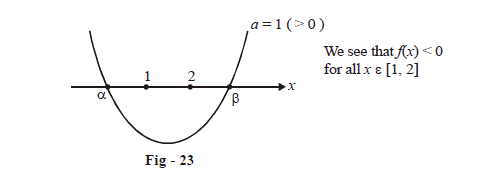This corresponds to “k1 and k2 lie between the roots”. The constraints are:

\begin{align}&a \cdot f\left( {{k_1}} \right) < 0\,\,\, \Rightarrow \,\,\,1 \cdot \left\{ {{{\left( 1 \right)}^2} + a \cdot \left( 1 \right) + {a^2} + 6a} \right\} < 0\\&\qquad\qquad\qquad\Rightarrow \,\,\,{a^2} + 7a + 1 < 0\\&\qquad\qquad\qquad\Rightarrow \,\,\,\frac{{ - 7 - 3\sqrt 5 }}{2} < a < \frac{{ - 7 + 3\sqrt 5 }}{2}\end{align}

\begin{align}&a \cdot f\left( {{k_2}} \right) < 0\,\,\, \Rightarrow \,\,\,1 \cdot \left\{ {{{\left( 2 \right)}^2} + a \cdot \left( 2 \right) + {a^2} + 6a} \right\} < 0\\&\qquad\qquad\qquad\Rightarrow \,\,\,{a^2} + 8a + 4 < 0\\&\qquad\qquad\qquad\Rightarrow \,\,\, - 4 - 2\sqrt 3 < a < - 4 + 2\sqrt 3\end{align}

We now find the values of a satisfying both the constraints above: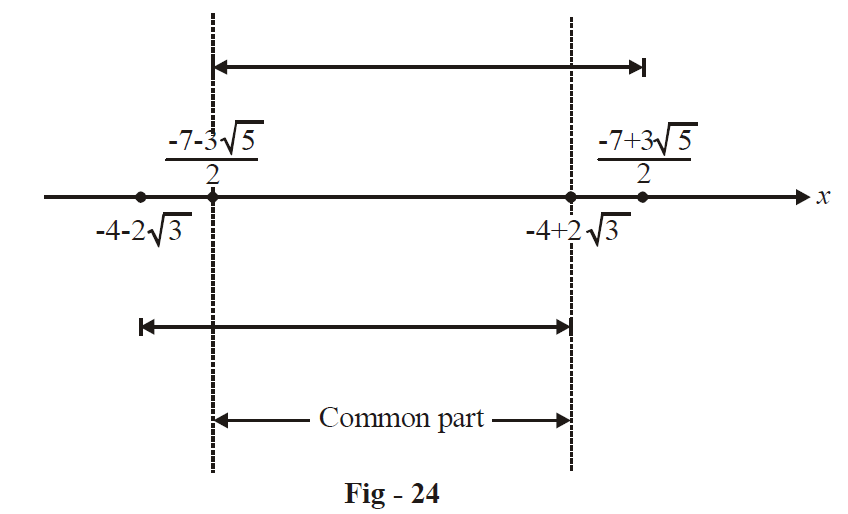We see that:

$\frac{{ - 7 - 3\sqrt 5 }}{2} < a < - 4 + 2\sqrt 3$

Example – 23

For what real values of a do the roots of the equation $$f\left( x \right) = {x^2} - 2x - \left( {{a^2} - 1} \right) = 0$$ lie between the roots of $$g\left( x \right) = {x^2} - 2\left( {a + 1} \right)x + a\left( {a - 1} \right) = 0?$$

Solution: The coefficients of  $${x^2}$$  in both  $$f\left( x \right)$$  and $$g\left( x \right)$$  are positive (both coefficients are 1) so the parabolas for both  $$f\left( x \right)\,\,{\rm{and}}\,\,g\left( x \right)$$ open upwards.

Since the roots of $$f\left( x \right)$$  lie between the roots of  $$g\left( x \right),$$ the parabola for $$f\left( x \right)$$  should lie “inside” the parabola for $$g\left( x \right);$$ observe the figure below: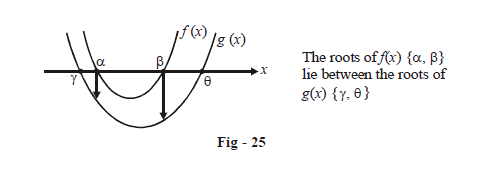We can now say that since $$\alpha ,\beta$$  lie between the roots of  $$g\left( x \right),$$

$\left. \begin{array}{l}g\left( \alpha \right) < 0\\g\left( \beta \right) < 0\end{array} \right\}{\rm{Section - 3,}}\,{\rm{part}}\left( {\rm{J}} \right)$

To solve the inequalities above, we need $$\alpha ,\beta$$ (the solution of  $$f\left( x \right) = 0$$ )

\begin{align}f\left( x \right) &= {x^2} - 2x - \left( {{a^2} - 1} \right)\\ &= {x^2} - 2x + 1 - {a^2}\\&= {\left( {x - 1} \right)^2} - {a^2} = 0\\ \Rightarrow\;\;\quad \left( {x - 1} \right) &= \pm \,a\\ \Rightarrow\qquad\qquad x &= 1 \pm \,a\end{align}

Let $$\alpha = 1 + a\,\,\,{\rm{and}}\,\,\,\beta = 1 - a$$

Now, we have:

\begin{align}&g\,\left( \alpha \right) < 0 \quad\Rightarrow\quad g\left( {1 + a} \right) < 0\\ &\qquad\qquad\quad\Rightarrow \quad {\left( {1 + a} \right)^2} - 2\left( {a + 1} \right)\left( {1 + a} \right) + a\left( {a - 1} \right) < 0\\ &\qquad\qquad\quad\Rightarrow\quad - {\left( {1 + a} \right)^2} + a\left( {a - 1} \right) < 0\\ &\qquad\qquad\quad\Rightarrow\quad - 3a - 1 < 0\\ &\qquad\qquad\quad\Rightarrow\quad 3a > - 1\\ &\qquad\qquad\quad\Rightarrow\quad a > - \frac{1}{3}\end{align}

\begin{align}&g\left( \beta \right) < 0 \quad\Rightarrow\quad g\left( {1 - a} \right) < 0\\&\qquad\quad\quad\;\;\Rightarrow\quad {\left( {1 - a} \right)^2} - 2\left( {1 + a} \right)\left( {1 - a} \right) + a\left( {a - 1} \right) < 0\\&\qquad\quad\;\;\;\;\;\Rightarrow\quad 4{a^2} - 3a - 1 < 0\\&\qquad\quad\quad\;\Rightarrow\;\quad - \frac{1}{4} < a < 1\end{align}

The values of a that satisfy both the constraints are:

$- \frac{1}{4} < a < 1$

Example - 24

Let a, b, c be real numbers. If  $$a{x^2} + bx + c = 0$$  has two real roots  $$\alpha ,\beta$$  where $$\alpha < - 1\,\,{\rm{and}}\,\,\beta > 1$$ then show that  $$1 + \frac{c}{a} + \left| {\frac{b}{a}} \right| < 0$$

Solution: We see that –1 and 1 lie between the roots of the equation. This scenario corresponds to Section-3 part (J).

The constraints that need to be satisfied are:

\begin{align}af\left( {{k_1}} \right) < 0 \quad &\Rightarrow\quad a \cdot \left\{ {a{{\left( 1 \right)}^2} + b\left( 1 \right) + c} \right\} < 0\\&\Rightarrow \quad a\left\{ {a + b + c} \right\} < 0\\&\qquad\text{Now we divide both sides of the inequality by } {a^2 } {\text{(this is allowed since } {a^2} > 0)}\\&\Rightarrow 1 + \frac{b}{a} + \frac{c}{a} < 0\;\;\;\;\; ... \rm{(i)}\end{align}

\begin{align}af\left( {{k_2}} \right) < 0 &\quad\Rightarrow \quad a \cdot \left\{ {a{{\left( { - 1} \right)}^2} + b\left( { - 1} \right) + c} \right\} < 0\\&\;\;\;\Rightarrow\quad a\left\{ {a - b + c} \right\} < 0\end{align}

Again, we divide both sides by $${a^2}$$ :

$\Rightarrow 1 - \frac{b}{a} + \frac{c}{a} < 0\;\;\;\;\; ... (ii)$

(i) and (ii) can be written simultaneously as:

$1 + \left| {\frac{b}{a}} \right| + \frac{c}{a} < 0$

Example - 25

Find the values of a for which the inequality

${4^x} - a\left( {{2^x}} \right) - a + 3 \le 0$

is satisfied by at least one real x.

Solution: Note that since  $${2^x} > 0$$, if we consider the quadratic inequality as a quadratic in $$y = {2^x}:$$

${y^2} - ay - a + 3 \le 0$

this must be satisfied for at least one positive y,  which means that both the roots of the equation.

${y^2} - ay - a + 3 = 0\;\;\;\;\;\;\; ...(1)$

cannot be non-positive

Now, (1) will have real roots if

${a^2} + 4(a - 3) \ge 0\;\;\;\;\;\; \Rightarrow\;\;\;\;\;\; a \in \left( { - \infty , - 6} \right] \cup \left[ {2,\;\infty } \right)$

Also, both roots are non-positive if

$a \le 0,\; - a + 3 \ge 0 \;\;\;\;\;\; \Rightarrow \;\;\;\;\;\; a \in \left( { - \infty , - 6} \right]$

Thus, for at least one positive root, we have $$a \in [2,\;\infty )$$

TRY YOURSELF – III

1.  Find  $$p \in \mathbb{R}$$ for which $${x^2} - px + p + 3 = 0$$  has

(a) one positive and one negative root

(b) both roots negative

(c) one root > 2 and one root < 2

2. If $${x^2} - \left( {m + 1} \right)x + {m^2} + m - 8 = 0$$ has one root in $$\left( {1,\;\infty } \right)$$  and the other in $$\left( { - \infty ,\;1} \right)$$ , find the possible values of m.

3.  For what values of  $$a\, \in \mathbb{R}{\text{}}$$ does the equation

$$\left( {{a^2} + 1} \right){x^2} - \left( {a + 1} \right)x + \left( {{a^2} - a - 2} \right) = 0$$

has one root positive and the other negative?

4.  For what values of a does the equation  $$a{x^2} - \left( {a + 1} \right)x + 3 = 0$$  has roots lying between 1 and 2?

5.  Find a such that $$\left( {2a + 1} \right){x^2} - a\left( {x - 1} \right) = 2$$ has one root greater than 1 and the other less than 1.

6. Find the values of k for which the equation

$${x^4} + \left( {1 - 2k} \right){x^2} + \left( {{k^2} - 1} \right) = 0$$

(a) has no realsolution

(b) has one real solution

Learn from the best math teachers and top your exams

• Live one on one classroom and doubt clearing
• Practice worksheets in and after class for conceptual clarity
• Personalized curriculum to keep up with school